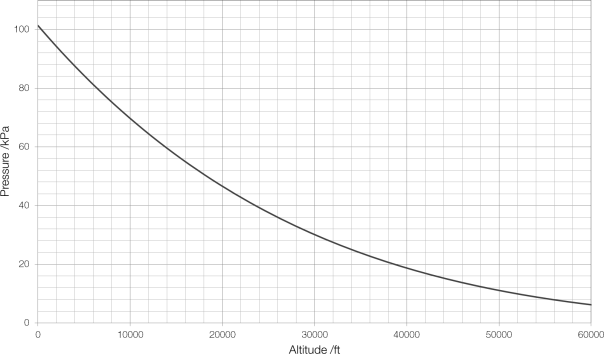# Altitude and Flight Level

The term elevation refers to the position of a point or an object on the ground above a fixed reference point, usually mean sea level. The term altitude refers to the position of a point or object in the air above a fixed reference point.

But defining altitude can be difficult, and so when altitude is referred to, the reference point must always be explicitly defined. Altitude is normally measured above mean sea level (AMSL) or above ground level (AGL). For example, if an aeroplane flew over the peak of Mount Everest, its altitude could be referred to as either 38 000 ft AMSL or 9000 ft AGL, because the peak of Everest has an elevation of 29 000 ft.

For aircraft it is difficult to measure altitude. You might think that GPS could provide this information, but GPS is designed for positioning on the surface of the Earth and isn’t very good at measuring altitude.

Pilots have always used atmospheric pressure to measure altitude. As an aircraft moves further upwards into the air, there is less air above it pushing down on it and the pressure decreases. This decrease is predictable and easy to calculate:$p_h = p_0 \left( 1- \frac{\gamma h}{T_0} \right)^{\frac{gM}{R\gamma}}$

where$p_h$ is the pressure at height$h$$p_0$ is the standard atmospheric pressure of 1013.25 hPa$\gamma$ is the rate at which temperature decreases with altitude (the temperature lapse rate);$T_0$ is the standard temperature;$g$ is the gravitational field strength;$M$ is the molar mass of dry air; and$R$ is the molar gas constant.Aeroplanes do not fly at a set altitude. Rather they fly at a given flight level, which – although it sounds like a height – is actually a pressure. When a pilot flies at a flight level of 32 000 ft (FL320) they are actually flying at a constant pressure of 275 hPa, and may actually be far above or far below this altitude, depending on the local weather (and therefore pressure) conditions. If they enter an area of particularly high or low pressure they will have to ascend or descend correspondingly.

Using flight levels helps to prevent collisions between aircraft: in the UK aircraft flying on headings between 000° and 089° (north to east) they flight at odd numbered flight levels (FL310, FL330, etc); flying between 090° and 179° (east to south) at odd numbered flight levels plus 500 ft (FL315, FL335); flying between 180° and 269° (south to west) at even numbered flight levels (FL320, FL340); and flying between 270° and 359° (west to north) at even numbered flight levels plus 500 ft (FL325, FL 345). In other parts of the world different flight level rules are used.